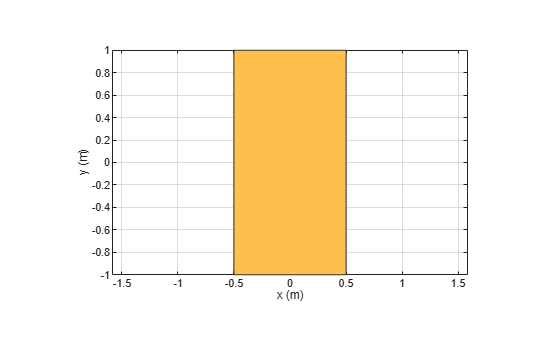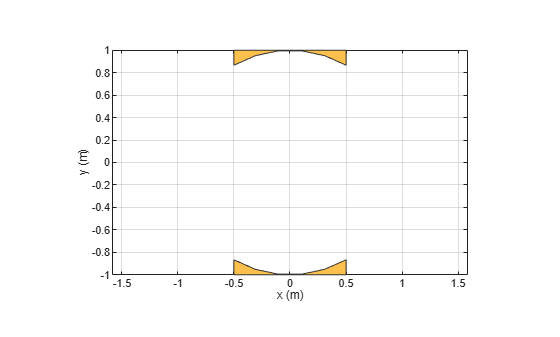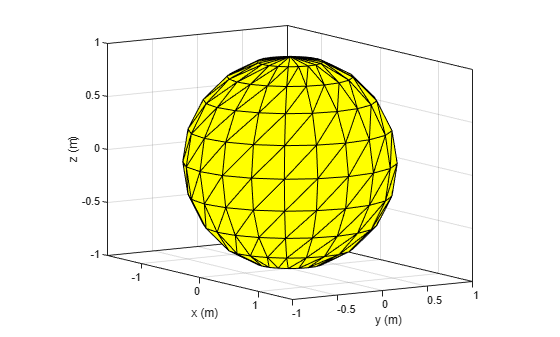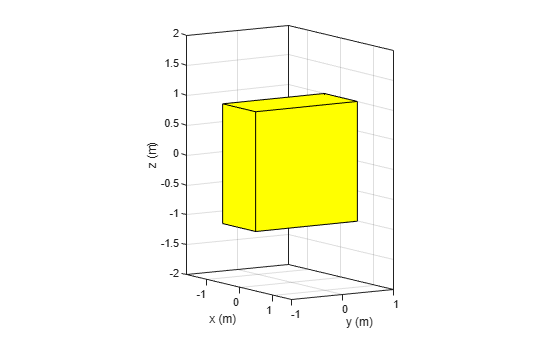# minus

Carve a shape from other shape

## Syntax

``minus(shape1,shape2)``
``c = minus(shape1,shape2)``

## Description

example

````minus(shape1,shape2)` carves out `shape2` from `shape1` and plots the resultant shape. Alternatively, you can also use the `'-'` operator to carve out a shape from other shape (`shape1 - shape2`).```

example

````c = minus(shape1,shape2)` generates the shape obtained by carving out `shape2` from `shape1` and returns a polygon object for resultant 2-D shape or a custom 3-D object for resultant 3-D shape. Alternatively, you can also use the `'-'` operator to carve out a shape from other shape (`shape1 - shape2`).```

## Examples

collapse all

Create and view a rectangular shape.

```r = antenna.Rectangle; show(r)```Create and view a circular shape.

```c = antenna.Circle; show(c)```Carve out the circle from the rectangle and view the resultant shape.

```s = r-c; show(s)```This example shows how to create a hollow sqaure nut shape by carving out a sphere from a box shape.

Create and view a spherical shape.

```a = shape.Sphere; show(a)```Create and view a box shape.

```b = shape.Box; show(b)```Carve out the sphere from the box and view the resultant shape.

`minus(b,a);`## Input Arguments

collapse all

2-D and 3-D geometric shapes created using shape objects in Custom 2-D and 3-D Antenna.

Example: `antenna.Rectangle,antenna.Circle`

Example: `shape.Box,shape.Sphere`

## Output Arguments

collapse all

2-D or 3-D shape obtained after carving out `shape2` from `shape1`.

Example: `antenna.Polygon`

Example: `shape.Polygon`

Example: `shape.Custom3D`

## Version History

Introduced in R2017a